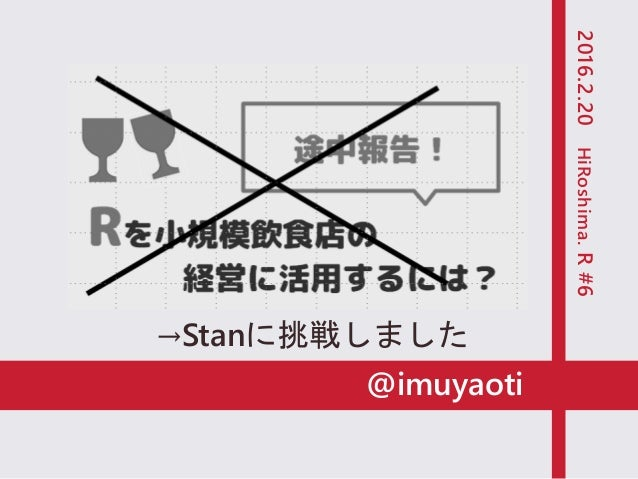Successfully reported this slideshow.Upcoming SlideShare
×

# HiRoshima.R#6 by imuyaoti

771 views

Published on

2016.2.20 HiRoshima.R#6 でimuyaotiが発表したものにスライド追加したものです。

とりあえずやってみた！という事例報告程度の内容なのでご注意ください。
モデルの試行錯誤や結果の解釈はほとんどできていません。

Published in: Data & Analytics
• Full Name
Comment goes here.

Are you sure you want to Yes No• Be the first to comment

### HiRoshima.R#6 by imuyaoti

1. 1. @imuyaoti Rを小規模飲食店の 経営に活用するには？ 2016.2.20HiRoshima.R#6 途中報告！ →Stanに挑戦しました
2. 2. 2 お店の売り上げデータ 好きにしていいよって 言われたけど、 Rで何かできないかな
3. 3. 3
4. 4. 4 こんなことが できるように なりたい！！
5. 5. データの概要 データ 5 ・期間：2015/3/18～2015/12/31 ・客数：合計、新規客 ・売上：合計、チャージ、ドリンク、フード ・注文数：合計、チャージ、ドリンク、フード ・その他：天候、気温、雨量 仕入や経費のデータは今回は扱わない
6. 6. 6 売上を上げる 方法を考えたい！
7. 7. 売上とは？ 7 売上 ドリンク売上 フード売上 チャージ売上 ＝ チャージ金額 × 客数 ＝ １人当たりドリンク平均額 × 客数 ＝ １人当たりフード平均額 × 客数 ドリンク平均単価×１人当たりドリンク注文数 フード平均単価×１人当たりフード注文数
8. 8. 売上とは？ 8 𝑺 𝒕 𝑺 𝒅 𝑺 𝒇 𝑺 𝒄 ＝ 𝑷 𝒄 × 𝑪𝒕𝒎 ＝ 𝑨 𝒅 × 𝑪𝒕𝒎 ＝ 𝑨 𝒇 × 𝑪𝒕𝒎 𝑷 𝒅 × 𝑵 𝒅 𝑷 𝒇 × 𝑵 𝒇 𝑺 ：売上、𝑪𝒕𝒎：客数、 𝑨 ：客単価、 𝑷 ：単価および平均単価、 𝑵 ：１人当たりの平均注文数、 𝒕 ：合計、 𝒄 ：チャージ、 𝒅 ：ドリンク、 𝒇 ：フード 数式にすると…
9. 9. 売上とは？ 9 𝑺 𝒕 = 𝑪𝒕𝒎 × 𝑷 𝒄 + 𝑷 𝒅 × 𝑵 𝒅 + 𝑷 𝒇 × 𝑵 𝒇 𝑨 𝒅 𝑨 𝒇 𝑨 𝒕 売上 ＝ 客数 × 客単価 つまりは 整理すると… 𝑺 𝒕 = 𝑪𝒕𝒎 × 𝑨 𝒕
10. 10. 売上とは？ 10 売上アップに効果的な要因はどれか？ 𝑺 𝒕 = 𝑪𝒕𝒎 × 𝑷 𝒄 + 𝑷 𝒅 × 𝑵 𝒅 + 𝑷 𝒇 × 𝑵 𝒇 お客さんを増やす？ チャージ金額を上げる？ 価格の高いメニューをすすめる？ 注文数を増やす？ 商品の価格を上げる？ いろいろあるけど…
11. 11. 11 わたしができることは グラスが空いているお客さんに 「もう一杯いかがですか？」 と声をかけることぐらい… 現実は... 𝑺 𝒕 = 𝑪𝒕𝒎 × 𝑷 𝒄 + 𝑷 𝒅 × 𝑵 𝒅 + 𝑷 𝒇 × 𝑵 𝒇 １人当たりのドリンク注文数を増やす
12. 12. • 売上予測モデルをつくる • 声掛けで増加できる𝑵 𝒅を推定する • 𝑵 𝒅が増加した場合の今後の売上を予測する 早くもココで挫折 声かけによるドリンク注文数の増加が 売上に及ぼす影響を予測したい
13. 13. どんなモデル？ 13 𝑪𝒕𝒎 = 𝑾𝒕𝒓 + 𝑴𝒕𝒉 + 𝑫𝒂𝒚 + 𝑯𝒐𝒍 … ：アテがあればお酒がススム 天候 月 曜日 休日 𝑵 𝒅 ∝ 𝑵 𝒇 とりあえず思いついたこと
14. 14. どんなモデル？ 時系列データである！ 14
15. 15. データをながめる 15 Data %>% ggplot(aes(x = ymd))+ geom_line(aes( y = sal), color= "#a9a9a9", size = 0.8)+ geom_line(aes( y = TTR：：SMA(sal, n = 7)), color= "#f8766D", size = 0.8)+ geom_hline(yintercept = mean(Data\$sal), color = "#dc143c", size = 0.5)+ labs(x = “Time”, y =“St：売上”)+ my.theme 3月開店 12月定休日なし ゆるやかに増加傾向？ 売上の推移
16. 16. データをながめる 16 売上と客数の傾向は類似 客単価は横ばい 売上・客数・客単価の推移を比較
17. 17. データをながめる 17 ggpairs{GGally} 客数と関連しそうな要因
18. 18. データをながめる 18 細かいところは見にくいですが、 これくらい全体をざっとながめて データの雰囲気を つかめるようになりたい
19. 19. データをながめる 19 客数と関連しそうな要因pick up 天候 休日前日 月 曜日 などなど。。。
20. 20. 20 ぜんぶ見てたら進めない… とりあえずで 何かつくってやってみよ…
21. 21. モデル作成 21 𝑺 𝒕 = 𝑪𝒕𝒎 × 𝑨 𝒕 𝑵 𝒇 = 𝜶 + 𝜷𝑵 𝒅 + 𝜺 𝟐 𝑨 𝒕 = 𝑨 𝒅 + 𝑨 𝒇 𝑪𝒕𝒎 = 𝑻𝒓𝒆𝒏𝒅 + 𝑹𝒂𝒊𝒏 + (𝑫𝒂𝒚 + 𝑷𝒉𝒐𝒍) + 𝑨𝑹 + 𝜺 𝟏 𝑨 𝒇 = 𝑷 𝒇 × 𝑵 𝒇 ・売上を客数と客単価から算出する ・客数を天候、曜日、休日前日効果、時系列的要因で予測する ・ドリンク注文数がフード注文数に影響する こんなモデルを考えてみた 時系列プロットの比較から 𝑺 𝒕の時間的変動は 𝑪𝒕𝒎によるものとした。 𝒘𝒆𝒆𝒌
22. 22. RStanでうごか… 22 いろいろとひどすぎて ぜんぜんできなかった
23. 23. 23 申し訳ないですが 今ここまでです。。 真似すればできるものではなかった… 大いに反省中。。。
24. 24. 24 ここから延長戦。 ・Stanの文法をちゃんと勉強する ・簡単なモデルからはじめて、 最後に組み合わせる で、Stanをうごかすことはできました。
25. 25. • 休日効果は休日前日効果のみ • イベント効果を入れていない • generated quantitiesブロックで 予測値を計算した 25 と少し違うところ
26. 26. data{ int<lower=0> N; # サンプルサイズ real<lower=0> Nd[N]; # １人当たりドリンク注文数 real<lower=0> Nf[N]; # １人当たりフード注文数 real<lower=0> Pd[N]; # ドリンク平均単価 real<lower=0> Pf[N]; # フード平均単価 int<lower=0, upper=6> Day[N]; # 曜日(0～6：日～土) int<lower=0, upper=1> Phol[N]; # 休日前日＝1、それ以外＝0 real<lower=0, upper=1> Rain[N]; # 雨量効果 (0~0.9mm = 0、 1.0~9.9mm = 0.5、 10.0~19.9mm = 0.5、20mm~ = 1) real<lower=0> Ctm[N]; # 客数 } data 26 ※オープン月の３月のデータは除いた
27. 27. parameters parameters{ real<lower=0> b1; real b2; real trend[N]; real<lower=0> b3; real<lower=0> b4; real s[N]; real c_rain[N]; real ar[N]; real c_ar; real<lower=0, upper=100> sigma; } 27
28. 28. model model{ real week[N]; real rain[N]; real ctm_mu[N]; for(i in 1:N) Nf[i] ~ normal(b1 + b2*Nd[i], sigma); for(i in 3:N) trend[i] ~ normal(2*trend[i-1] - trend[i-2], sigma); for(i in 7:N) s[i] ~ normal(-s[i-1]-s[i-2]-s[i-3]-s[i-4]-s[i-5]-s[i-6], sigma); for(i in 1:4) # データが水曜 始まりのため week[i] <- s[i]; for(i in 5:N) week[i] <- s[i] + Phol[i]*(b3*(s[i-Day[i]-2]-s[i])+b4*(s[i-Day[i]-1]-s[i])); for(i in 1:N) rain[i] <- c_rain*Rain[i]; for(i in 3:N) ar[i] ~ normal(c_ar*ar[i-1] + c_ar*ar[i-2], sigma); for(i in 1:N) ctm_mu[i] <- trend[i] + week[i] + ar[i]; for(i in 1:N) Ctm[i] ~ normal(ctm_mu[i], sigma); 28 𝒑𝒂𝒓𝒂𝒎𝒆𝒕𝒂𝒓𝒔の推定に必要な、 計算によって求められる変数の宣言 ※generated quantitiesに引き継げない！
29. 29. generated quantities generated quantities{ real nf_pre[N]; real at_pre[N]; real week2[N]; real rain2[N]; real ctm_mu2[N]; real ctm_pre[N]; real sal_pre[N]; for(i in 1:N) nf_pre[i] <- normal_rng(b1 + b2*Nd[i], sigma); for(i in 1:N) at_pre[i] <- Pd[i]*Nd[i] + Pf[i]*nf_pre[i] + 500; for(i in 1:4) week2[i] <- s[i]; for(i in 5:N) week2[i] <- s[i] + Phol[i]*(b3*(s[i-Day[i]-2]-s[i])+b4*(s[i-Day[i]-1]-s[i])); for(i in 1:N) ctm_mu2[i] <- trend[i] + week2[i] + ar[i]; for(i in 1:N) ctm_pre[i] <- normal_rng(ctm_mu2[i], sigma); for(i in 1:N) sal_pre[i] <- ctm_pre[i]*at_pre[i]; 29 𝒎𝒐𝒅𝒆𝒍 から引き継げないので、 変数名を変えて再定義
30. 30. kick library(rstan) war <- 1000 ite <- 10000 dig <- 3 cha <- 3 Thi <- 30 rstan_options(auto_write = TRUE) options(mc.cores = parallel::detectCores()) data <- list(N=nrow(Data), Nd = Data\$nd, Nf = Data\$nf, Pf = Data\$pf, Pd = Data\$pd, Day=day, Phol = Data2\$phol, Rain = Data2\$rain, Ctm = Data2\$ctm) fit <- stan(file = ".stan", data = data, thin = thi, verbose = F, chains = cha, warmup = war, iter = ite) 30
31. 31. 結果：客数の成分分解 31 𝒘𝒆𝒆𝒌𝑻𝒓𝒆𝒏𝒅 𝑹𝒂𝒊𝒏 𝑨𝑹
32. 32. 結果：客数…週効果 32 b3：直近の金曜日との類似度 b4：直近の土曜日との類似度 𝑫𝒂𝒚 𝑷𝒉𝒐𝒍
33. 33. 結果：客数…雨効果 33 𝑪_𝑹𝒂𝒊𝒏 ・仮説検定的に考えると、 信頼区間がゼロをまたいでいる ・値でみても、どしゃぶり20㎜以上の日 でも1名程度しか客数に影響はない
34. 34. 結果 客単価、フード注文数 34 𝑵𝒇 = 𝒃𝟏 + 𝒃𝟐 ∗ 𝑵𝒅 + 𝜺 青：実測値 赤：予測値 𝑵 𝒇 青：実測値 赤：予測値 単純にドリンク注文数で予測できるものではなかった
35. 35. 結果：観測値と予測値の比較 35 𝑺 𝒕 𝑪𝒕𝒎 𝑨 𝒕 客単価に占めるフードの割合が小さく、 フード注文数の予測が悪くても 客単価予測にあまり影響しない。 青：実測値 赤：予測値 青：実測値 赤：予測値 青：実測値 赤：予測値
36. 36. 参考にさせていただきました @berobero11さんのスライド RStanで『予測にいかす統計モデリングの基本』 の売上データの分析をトレースしてみた http://www.slideshare.net/berobero11/ss-29409382 36 樋口知之先生 著 予測にいかす統計モデリングの基本 牛 廿TTさんのページ 状態空間モデルで自然検索トラフィックの成長を 予測する http://abrahamcow.hatenablog.com/entry/2016/02/06/080906 などなど
37. 37. 参考にさせていただきました Masaki Tsudaさんのスライド RStanとShinyStanによるベイズ統計モデリング 入門http://www.slideshare.net/masakitsuda940/rstanshinystan 37 豊田秀樹先生 編著 基礎からのベイズ統計学 ハミルトニアンモンテカルロ法による実践的入門 馬場真哉さんのページ Logics of Blue Stanによるベイズ推定の基礎 http://logics-of-blue.com/stanによるベイズ推定の基礎/ などなど